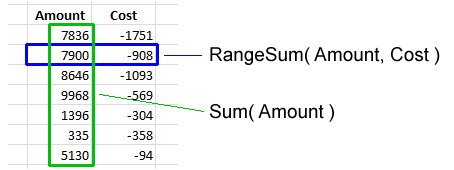# Qlik Design Blog

All about product and Qlik solutions: scripting, data modeling, visual design, extensions, best practices, etc.Employee

## It’s all Aggregations

I often see incorrect expressions being used in the QlikCommunity forum. Expressions that seem to work correctly – but really don’t…

So, let me make this clear: Calculations in QlikView are aggregations.

It doesn’t matter if it is a measure in a chart, or a calculated object label, or a show condition for an object, or a calculated color, or an expression search – all expressions in the user interface are evaluated as aggregations. (Except calculated dimensions, and some search strings.)

This means that it is correct to use the Sum() function in an expression, since this is an aggregation function - a function that uses several records as input. But if you omit the aggregation function or use a scalar function only, e.g. RangeSum(), you can get an unexpected behavior.Basically, all field references should be wrapped in an aggregation function. The Aggr() function and some constructions using the total qualifier can even have several layers of aggregations.

But if the created expression does not contain an aggregation function, the expression is ill-formed and potentially incorrect.

Examples:

=Sum(Amount)
=Count(OrderID)
These are both correct aggregations. Amount is wrapped in the Sum() function which will sum several records of the field Amount. OrderID is wrapped in the Count() function, which will count the records where OrderID has a value.

=Only(OrderID)
This is also a correct aggregation. OrderID is wrapped in the Only() function, which will return the OrderID if there is only one value, otherwise NULL.

=OrderID
A single field reference is not an aggregation, so this is an ill-formed expression. But QlikView will not throw an error. Instead it will use the Only() function to interpret the field reference. I.e., if there is only one value, this value will be used. But if there are several possible values, NULL will be used. So, it depends on the circumstances whether an expression without aggregation function is correct or not.

=If(Year=Year(Today()), Sum(Amount1), Sum(Amount2))
Here, both the amounts are correctly wrapped in the Sum() function. But the first parameter of the if() function, the condition, is not. Hence, this is an ill-formed expression. If it is used in a place where there are several possible Years, the field reference will evaluate to NULL and the condition will be evaluated as FALSE, which is not what you want. Instead, you probably want to wrap the Year in the Min() or Max() function.

=ProductGroup= 'Shoes'
=IsNull(ProductGroup)
These expressions can both be used as show conditions or as advanced searches. However, since there are no aggregation functions, the expressions are ill-formed. If you want to test whether there exists Shoes or NULL values among the field values, you probably want to use the following instead:
=Count(If(ProductGroup= 'Shoes', ProductGroup))>0
=NullCount(ProductGroup)>0

Conclusions:

• An aggregation function is a function that returns a single value describing some property of several records in the data.
• All UI expressions, except calculated dimensions, are evaluated as aggregations.
• All field references in expressions must be wrapped in an aggregation function. If they aren’t, QlikView will use the Only() function.

HIC

Further reading related to this topic:

The Only Function

Use Aggregation Functions!